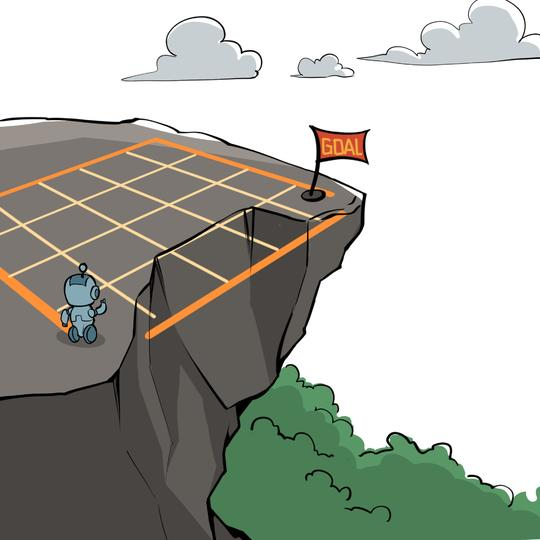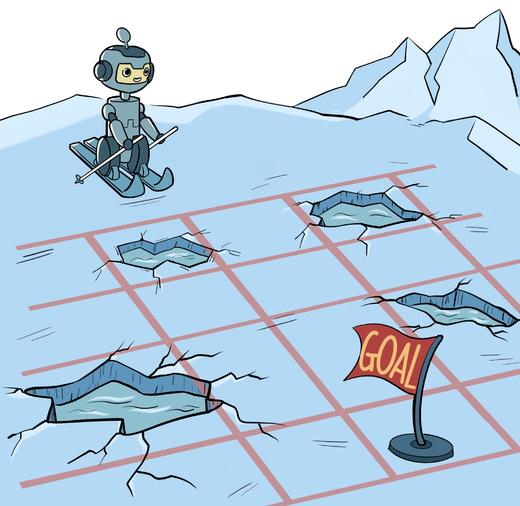# 第 4 章 动态规划算法

## 4.2 悬崖漫步环境import copy

class CliffWalkingEnv:    """ 悬崖漫步环境"""    def __init__(self, ncol=12, nrow=4):        self.ncol = ncol  # 定义网格世界的列        self.nrow = nrow  # 定义网格世界的行        # 转移矩阵P[state][action] = [(p, next_state, reward, done)]包含下一个状态和奖励        self.P = self.createP()
def createP(self):        # 初始化        P = [[[] for j in range(4)] for i in range(self.nrow * self.ncol)]        # 4种动作, change:上,change:下, change:左, change:右。坐标系原点(0,0)        # 定义在左上角        change = [[0, -1], [0, 1], [-1, 0], [1, 0]]        for i in range(self.nrow):            for j in range(self.ncol):                for a in range(4):                    # 位置在悬崖或者目标状态,因为无法继续交互,任何动作奖励都为0                    if i == self.nrow - 1 and j > 0:                        P[i * self.ncol + j][a] = [(1, i * self.ncol + j, 0,                                                    True)]                        continue                    # 其他位置                    next_x = min(self.ncol - 1, max(0, j + change[a]))                    next_y = min(self.nrow - 1, max(0, i + change[a]))                    next_state = next_y * self.ncol + next_x                    reward = -1                    done = False                    # 下一个位置在悬崖或者终点                    if next_y == self.nrow - 1 and next_x > 0:                        done = True                        if next_x != self.ncol - 1:  # 下一个位置在悬崖                            reward = -100                    P[i * self.ncol + j][a] = [(1, next_state, reward, done)]        return P

## 4.3 策略迭代算法

### 4.3.3 策略迭代算法

• 随机初始化策略 和价值函数
• while do : （策略评估循环）
• 对于每一个状态 :
• end while
• 对于每一个状态 :
• , 则停止算法并返回 ; 否则转到策略评估循环

class PolicyIteration:    """ 策略迭代算法 """    def __init__(self, env, theta, gamma):        self.env = env        self.v =  * self.env.ncol * self.env.nrow  # 初始化价值为0        self.pi = [[0.25, 0.25, 0.25, 0.25]                   for i in range(self.env.ncol * self.env.nrow)]  # 初始化为均匀随机策略        self.theta = theta  # 策略评估收敛阈值        self.gamma = gamma  # 折扣因子
def policy_evaluation(self):  # 策略评估        cnt = 1  # 计数器        while 1:            max_diff = 0            new_v =  * self.env.ncol * self.env.nrow            for s in range(self.env.ncol * self.env.nrow):                qsa_list = []  # 开始计算状态s下的所有Q(s,a)价值                for a in range(4):                    qsa = 0                    for res in self.env.P[s][a]:                        p, next_state, r, done = res                        qsa += p * (r + self.gamma * self.v[next_state] * (1 - done))                        # 本章环境比较特殊,奖励和下一个状态有关,所以需要和状态转移概率相乘                    qsa_list.append(self.pi[s][a] * qsa)                new_v[s] = sum(qsa_list)  # 状态价值函数和动作价值函数之间的关系                max_diff = max(max_diff, abs(new_v[s] - self.v[s]))            self.v = new_v            if max_diff < self.theta: break  # 满足收敛条件,退出评估迭代            cnt += 1        print("策略评估进行%d轮后完成" % cnt)
def policy_improvement(self):  # 策略提升        for s in range(self.env.nrow * self.env.ncol):            qsa_list = []            for a in range(4):                qsa = 0                for res in self.env.P[s][a]:                    p, next_state, r, done = res                    qsa += p * (r + self.gamma * self.v[next_state] * (1 - done))                qsa_list.append(qsa)            maxq = max(qsa_list)            cntq = qsa_list.count(maxq)  # 计算有几个动作得到了最大的Q值            # 让这些动作均分概率            self.pi[s] = [1 / cntq if q == maxq else 0 for q in qsa_list]        print("策略提升完成")        return self.pi
def policy_iteration(self):  # 策略迭代        while 1:            self.policy_evaluation()            old_pi = copy.deepcopy(self.pi)  # 将列表进行深拷贝,方便接下来进行比较            new_pi = self.policy_improvement()            if old_pi == new_pi: break

def print_agent(agent, action_meaning, disaster=[], end=[]):    print("状态价值：")    for i in range(agent.env.nrow):        for j in range(agent.env.ncol):            # 为了输出美观,保持输出6个字符            print('%6.6s' % ('%.3f' % agent.v[i * agent.env.ncol + j]), end=' ')        print()
print("策略：")    for i in range(agent.env.nrow):        for j in range(agent.env.ncol):            # 一些特殊的状态,例如悬崖漫步中的悬崖            if (i * agent.env.ncol + j) in disaster:                print('****', end=' ')            elif (i * agent.env.ncol + j) in end:  # 目标状态                print('EEEE', end=' ')            else:                a = agent.pi[i * agent.env.ncol + j]                pi_str = ''                for k in range(len(action_meaning)):                    pi_str += action_meaning[k] if a[k] > 0 else 'o'                print(pi_str, end=' ')        print()

env = CliffWalkingEnv()action_meaning = ['^', 'v', '<', '>']theta = 0.001gamma = 0.9agent = PolicyIteration(env, theta, gamma)agent.policy_iteration()print_agent(agent, action_meaning, list(range(37, 47)), )
策略评估进行60轮后完成策略提升完成策略评估进行72轮后完成策略提升完成策略评估进行44轮后完成策略提升完成策略评估进行12轮后完成策略提升完成策略评估进行1轮后完成策略提升完成状态价值：-7.712 -7.458 -7.176 -6.862 -6.513 -6.126 -5.695 -5.217 -4.686 -4.095 -3.439 -2.710-7.458 -7.176 -6.862 -6.513 -6.126 -5.695 -5.217 -4.686 -4.095 -3.439 -2.710 -1.900-7.176 -6.862 -6.513 -6.126 -5.695 -5.217 -4.686 -4.095 -3.439 -2.710 -1.900 -1.000-7.458  0.000  0.000  0.000  0.000  0.000  0.000  0.000  0.000  0.000  0.000  0.000策略：ovo> ovo> ovo> ovo> ovo> ovo> ovo> ovo> ovo> ovo> ovo> ovooovo> ovo> ovo> ovo> ovo> ovo> ovo> ovo> ovo> ovo> ovo> ovooooo> ooo> ooo> ooo> ooo> ooo> ooo> ooo> ooo> ooo> ooo> ovoo^ooo **** **** **** **** **** **** **** **** **** **** EEEE

## 4.4 价值迭代算法

• 随机初始化
• while do :
• 对于每一个状态 :
• end while
• 返回一个确定性策略

class ValueIteration:    """ 价值迭代算法 """    def __init__(self, env, theta, gamma):        self.env = env        self.v =  * self.env.ncol * self.env.nrow  # 初始化价值为0        self.theta = theta  # 价值收敛阈值        self.gamma = gamma        # 价值迭代结束后得到的策略        self.pi = [None for i in range(self.env.ncol * self.env.nrow)]
def value_iteration(self):        cnt = 0        while 1:            max_diff = 0            new_v =  * self.env.ncol * self.env.nrow            for s in range(self.env.ncol * self.env.nrow):                qsa_list = []  # 开始计算状态s下的所有Q(s,a)价值                for a in range(4):                    qsa = 0                    for res in self.env.P[s][a]:                        p, next_state, r, done = res                        qsa += p * (r + self.gamma * self.v[next_state] * (1 - done))                    qsa_list.append(qsa)  # 这一行和下一行代码是价值迭代和策略迭代的主要区别                new_v[s] = max(qsa_list)                max_diff = max(max_diff, abs(new_v[s] - self.v[s]))            self.v = new_v            if max_diff < self.theta: break  # 满足收敛条件,退出评估迭代            cnt += 1        print("价值迭代一共进行%d轮" % cnt)        self.get_policy()
def get_policy(self):  # 根据价值函数导出一个贪婪策略        for s in range(self.env.nrow * self.env.ncol):            qsa_list = []            for a in range(4):                qsa = 0                for res in self.env.P[s][a]:                    p, next_state, r, done = res                    qsa += p * (r + self.gamma * self.v[next_state] * (1 - done))                qsa_list.append(qsa)            maxq = max(qsa_list)            cntq = qsa_list.count(maxq)  # 计算有几个动作得到了最大的Q值            # 让这些动作均分概率            self.pi[s] = [1 / cntq if q == maxq else 0 for q in qsa_list]

env = CliffWalkingEnv()action_meaning = ['^', 'v', '<', '>']theta = 0.001gamma = 0.9agent = ValueIteration(env, theta, gamma)agent.value_iteration()print_agent(agent, action_meaning, list(range(37, 47)), )
价值迭代一共进行14轮状态价值：-7.712 -7.458 -7.176 -6.862 -6.513 -6.126 -5.695 -5.217 -4.686 -4.095 -3.439 -2.710-7.458 -7.176 -6.862 -6.513 -6.126 -5.695 -5.217 -4.686 -4.095 -3.439 -2.710 -1.900-7.176 -6.862 -6.513 -6.126 -5.695 -5.217 -4.686 -4.095 -3.439 -2.710 -1.900 -1.000-7.458  0.000  0.000  0.000  0.000  0.000  0.000  0.000  0.000  0.000  0.000  0.000策略：ovo> ovo> ovo> ovo> ovo> ovo> ovo> ovo> ovo> ovo> ovo> ovooovo> ovo> ovo> ovo> ovo> ovo> ovo> ovo> ovo> ovo> ovo> ovooooo> ooo> ooo> ooo> ooo> ooo> ooo> ooo> ooo> ooo> ooo> ovoo^ooo **** **** **** **** **** **** **** **** **** **** EEEE

## 4.5 冰湖环境import gymenv = gym.make("FrozenLake-v0")  # 创建环境env = env.unwrapped  # 解封装才能访问状态转移矩阵Penv.render()  # 环境渲染,通常是弹窗显示或打印出可视化的环境
holes = set()ends = set()for s in env.P:    for a in env.P[s]:        for s_ in env.P[s][a]:            if s_ == 1.0:  # 获得奖励为1,代表是目标                ends.add(s_)            if s_ == True:                holes.add(s_)holes = holes - endsprint("冰洞的索引:", holes)print("目标的索引:", ends)
for a in env.P:  # 查看目标左边一格的状态转移信息    print(env.P[a])
SFFFFHFHFFFHHFFG冰洞的索引: {11, 12, 5, 7}目标的索引: {15}[(0.3333333333333333, 10, 0.0, False), (0.3333333333333333, 13, 0.0, False), (0.3333333333333333, 14, 0.0, False)][(0.3333333333333333, 13, 0.0, False), (0.3333333333333333, 14, 0.0, False), (0.3333333333333333, 15, 1.0, True)][(0.3333333333333333, 14, 0.0, False), (0.3333333333333333, 15, 1.0, True), (0.3333333333333333, 10, 0.0, False)][(0.3333333333333333, 15, 1.0, True), (0.3333333333333333, 10, 0.0, False), (0.3333333333333333, 13, 0.0, False)]

# 这个动作意义是Gym库针对冰湖环境事先规定好的action_meaning = ['<', 'v', '>', '^']theta = 1e-5gamma = 0.9agent = PolicyIteration(env, theta, gamma)agent.policy_iteration()print_agent(agent, action_meaning, [5, 7, 11, 12], )
策略评估进行25轮后完成策略提升完成策略评估进行58轮后完成策略提升完成状态价值： 0.069  0.061  0.074  0.056 0.092  0.000  0.112  0.000 0.145  0.247  0.300  0.000 0.000  0.380  0.639  0.000策略：<ooo ooo^ <ooo ooo^<ooo **** <o>o ****ooo^ ovoo <ooo ******** oo>o ovoo EEEE

action_meaning = ['<', 'v', '>', '^']theta = 1e-5gamma = 0.9agent = ValueIteration(env, theta, gamma)agent.value_iteration()print_agent(agent, action_meaning, [5, 7, 11, 12], )
价值迭代一共进行60轮状态价值： 0.069  0.061  0.074  0.056 0.092  0.000  0.112  0.000 0.145  0.247  0.300  0.000 0.000  0.380  0.639  0.000策略：<ooo ooo^ <ooo ooo^<ooo **** <o>o ****ooo^ ovoo <ooo ******** oo>o ovoo EEEE

## 4.8 参考文献

 GERAMIFARD A, WALSN T J, TELLEX S, et al. A tutorial on linear function approximators for dynamic programming and reinforcement learning [J]. Foundations and Trends®in Machine Learning, 2013, 6 (4): 375-451.

 SZEPESVÁRI C, LITTMAN M L. Generalized markov decision processes: dynamic-programming and reinforcement-learning algorithms [C]//International Conference of Machine Learning, 1996: 96.